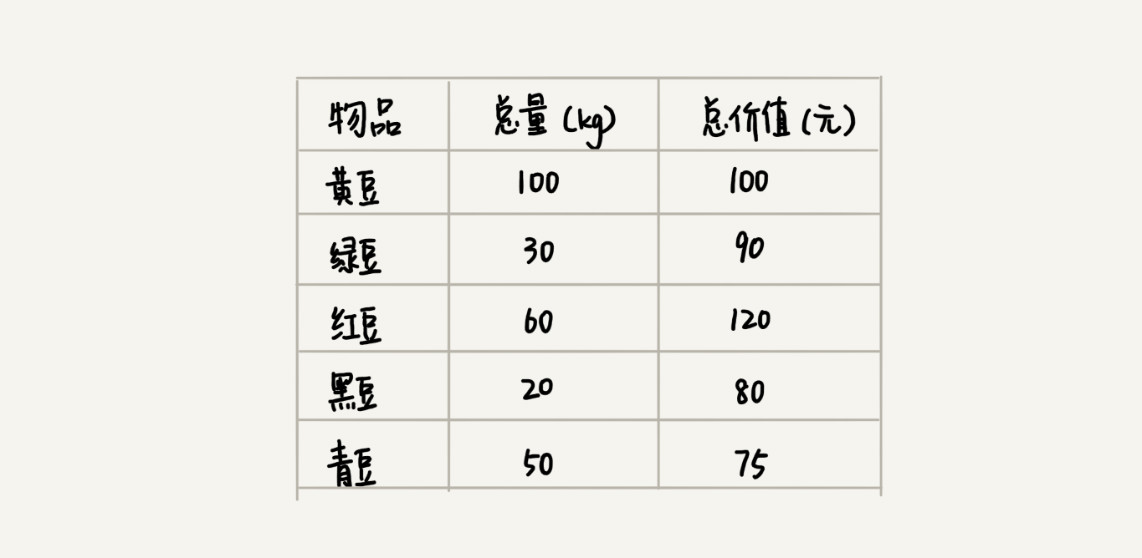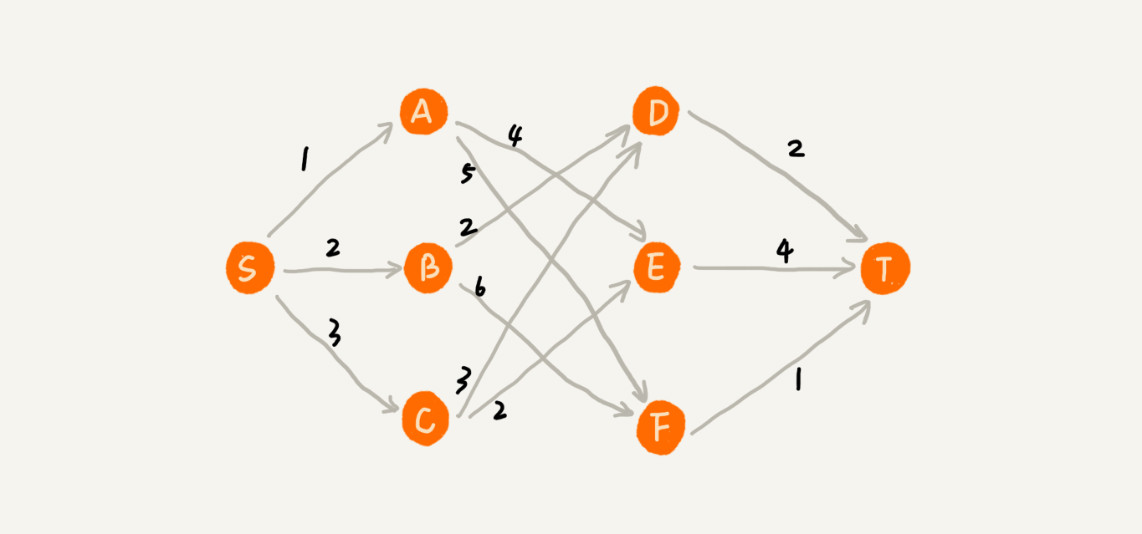## 一、基本概念

（即某个状态以后的过程不会影响以前的状态，只与当前状态有关。）

## 一、基本概念

（即某个状态以后的过程不会影响以前的状态，只与当前状态有关。）

## 六、贪心算法的实现框架

while (朝给定总目标前进一步)
{

}

## 七、题

### 1.背包问题

【背包问题】有一个背包，容量是M=150，有7个物品，物品可以分割成任意大小。要求尽可能让装入背包中的物品总价值最大，但不能超过总容量。

（1）根据贪心的策略，每次挑选价值最大的物品装入背包，得到的结果是否最优？
（2）每次挑选所占重量最小的物品装入是否能得到最优解？
（3）每次选取单位重量价值最大的物品，成为解本题的策略

W=30

（2）贪心策略：选取重量最小。它的反例与第一种策略的反例差不多。

（3）贪心策略：选取单位重量价值最大的物品。反例：

W=30### 2.分发糖果（Leetcode题目）

B

ratings
A

A

ratings
B

i

ratings
i−1

，则第 ii 名学生糖比第 i - 1i−1 名学生多 11 个。

i

<=ratings
i−1

，则第 ii 名学生糖数量不变。（交由从右向左遍历时处理。）

c++:

``````class Solution {
public:
int candy(vector<int>& ratings) {
int res=ratings.size();
vector<int> tmp(res,0);
for(int i=1;i<res;i++)
if(ratings[i]>ratings[i-1])
tmp[i]=tmp[i-1]+1;
for(int i=res-1;i>0;i--)
if(ratings[i-1]>ratings[i] && tmp[i-1]<tmp[i]+1)
tmp[i-1]=tmp[i]+1;
for(int i=0;i<ratings.size();i++)
res+=tmp[i];
return res;
}
};
``````

### 3. 钱币找零（有面值为1的可以”考虑”贪心，没有就 DP ）

C++：

``````#include<cstdio>
using namespace std;
int solve(int A);
const int v = {1,5,10,50,100,500};//面额
int main()
{
solve(620);
return 0;
}
int solve(int A)
{
int sum = 0;
int c = {3,2,1,3,0,2};//每种硬币的枚数
for(int i = 0 ; i<6 ; i++) //判断所有硬币的总额
sum += v[i]*c[i];
if(sum < A)
{
printf("总额不够");
return 0;
}
int temp,index,count = 0;
while(A != 0)
{
int min = 1000000;
for(int i = 0 ; i<6 ; i++)
{
if(A/v[i]<min&&A/v[i]>0&&c[i]>0)//Min表示枚数
{
min = A/v[i];//这儿有个问题，如果枚数大于剩余的枚数，下面的c[index]就成负数了，减多了
temp = v[i];
index = i;
}
}
count+=min;
c[index]-=min;
A = A-min*temp;
printf("%d\t",temp);
if(c[index]<0)              //把上面减多的加回来
{
A += temp*(-c[index]);
count+=c[index];
}

}
printf("count:%d",count);
return 0;
}
``````